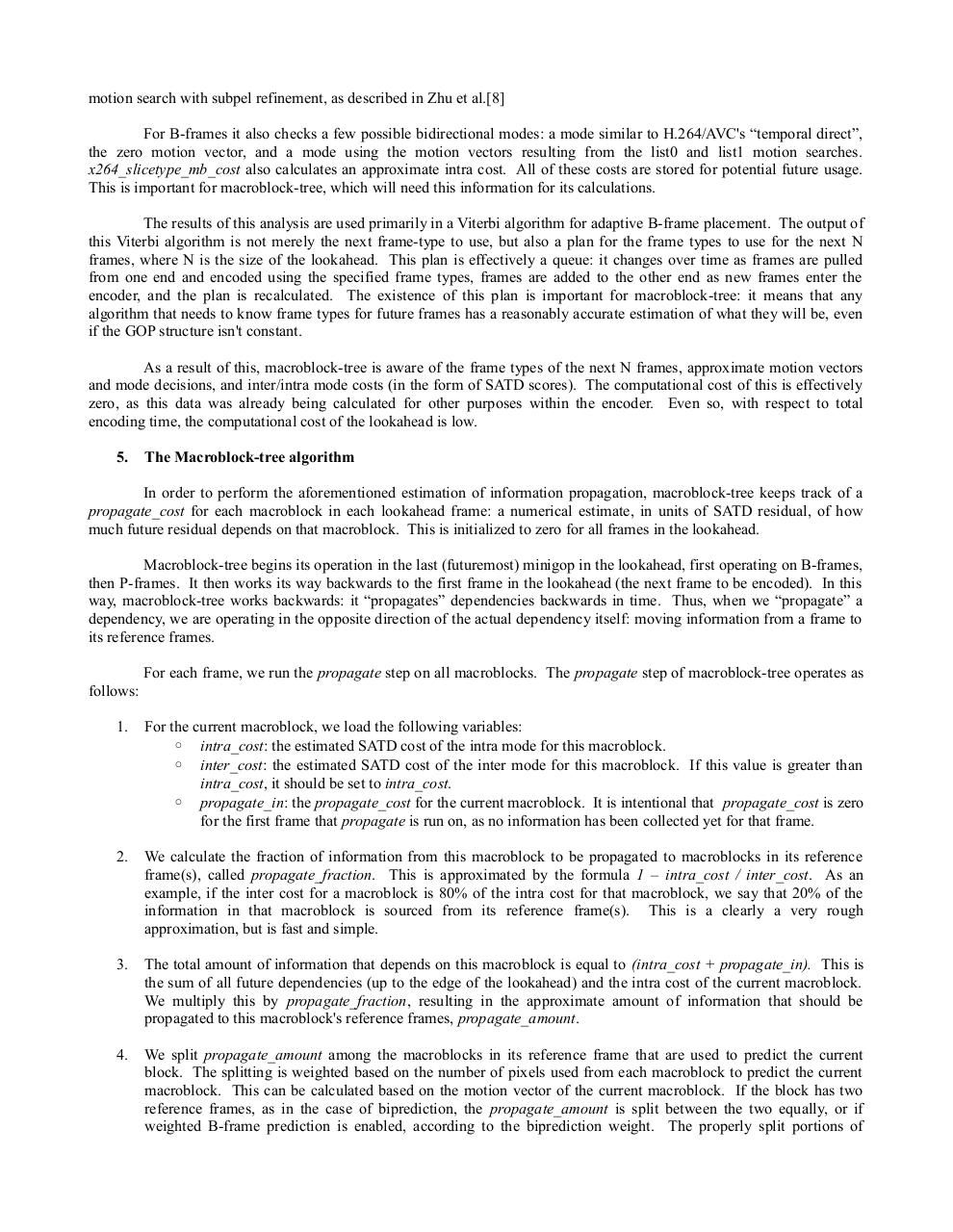# MBtree paper.pdfPage 1 2 3 4 5 6 7 8 9 10 11 12

#### Text preview

motion search with subpel refinement, as described in Zhu et al.
For B-frames it also checks a few possible bidirectional modes: a mode similar to H.264/AVC's “temporal direct”,
the zero motion vector, and a mode using the motion vectors resulting from the list0 and list1 motion searches.
x264_slicetype_mb_cost also calculates an approximate intra cost. All of these costs are stored for potential future usage.
This is important for macroblock-tree, which will need this information for its calculations.
The results of this analysis are used primarily in a Viterbi algorithm for adaptive B-frame placement. The output of
this Viterbi algorithm is not merely the next frame-type to use, but also a plan for the frame types to use for the next N
frames, where N is the size of the lookahead. This plan is effectively a queue: it changes over time as frames are pulled
from one end and encoded using the specified frame types, frames are added to the other end as new frames enter the
encoder, and the plan is recalculated. The existence of this plan is important for macroblock-tree: it means that any
algorithm that needs to know frame types for future frames has a reasonably accurate estimation of what they will be, even
if the GOP structure isn't constant.
As a result of this, macroblock-tree is aware of the frame types of the next N frames, approximate motion vectors
and mode decisions, and inter/intra mode costs (in the form of SATD scores). The computational cost of this is effectively
zero, as this data was already being calculated for other purposes within the encoder. Even so, with respect to total
encoding time, the computational cost of the lookahead is low.
5.

The Macroblock-tree algorithm

In order to perform the aforementioned estimation of information propagation, macroblock-tree keeps track of a
propagate_cost for each macroblock in each lookahead frame: a numerical estimate, in units of SATD residual, of how
much future residual depends on that macroblock. This is initialized to zero for all frames in the lookahead.
Macroblock-tree begins its operation in the last (futuremost) minigop in the lookahead, first operating on B-frames,
then P-frames. It then works its way backwards to the first frame in the lookahead (the next frame to be encoded). In this
way, macroblock-tree works backwards: it “propagates” dependencies backwards in time. Thus, when we “propagate” a
dependency, we are operating in the opposite direction of the actual dependency itself: moving information from a frame to
its reference frames.
For each frame, we run the propagate step on all macroblocks. The propagate step of macroblock-tree operates as
follows:
1.

For the current macroblock, we load the following variables:
◦ intra_cost: the estimated SATD cost of the intra mode for this macroblock.
◦ inter_cost: the estimated SATD cost of the inter mode for this macroblock. If this value is greater than
intra_cost, it should be set to intra_cost.
◦ propagate_in: the propagate_cost for the current macroblock. It is intentional that propagate_cost is zero
for the first frame that propagate is run on, as no information has been collected yet for that frame.

2.

We calculate the fraction of information from this macroblock to be propagated to macroblocks in its reference
frame(s), called propagate_fraction. This is approximated by the formula 1 – intra_cost / inter_cost. As an
example, if the inter cost for a macroblock is 80% of the intra cost for that macroblock, we say that 20% of the
information in that macroblock is sourced from its reference frame(s). This is a clearly a very rough
approximation, but is fast and simple.

3.

The total amount of information that depends on this macroblock is equal to (intra_cost + propagate_in). This is
the sum of all future dependencies (up to the edge of the lookahead) and the intra cost of the current macroblock.
We multiply this by propagate_fraction, resulting in the approximate amount of information that should be
propagated to this macroblock's reference frames, propagate_amount.

4.

We split propagate_amount among the macroblocks in its reference frame that are used to predict the current
block. The splitting is weighted based on the number of pixels used from each macroblock to predict the current
macroblock. This can be calculated based on the motion vector of the current macroblock. If the block has two
reference frames, as in the case of biprediction, the propagate_amount is split between the two equally, or if
weighted B-frame prediction is enabled, according to the biprediction weight. The properly split portions of